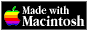THE HYPERCARD CENTER

 HyperTalk Reference

# subtract

`subtract number from `[`chunk`` of`]` ¬ container `where container or chunk must contain a number.

The` subtract `command subtracts` number `from the specified container (or chunk) and puts the result into the container (or chunk), replacing what was there.

(You can use the` is a `operator to see if the container is a number.)

For example, if you say

```subtract 3 from theTotal ``` and` theTotal `previously held 7, it will now hold 4.

## Examples

```subtract 4 from bkgnd field "total" subtract last line of card field "debits" from item 1 of theTotal if the Message box is a number then subtract 15 from Message box```

## Placeholders

number
An expression that evaluates to a number.

For example:

```169 / 19 pi (60.625 * 500) average(1,4,5,10) i+1 -- (where i is a number) line 1 of fld "Debits"```

Note: Formally, HyperCard distinguishes between factors (simple values) and expressions. The difference between factors and expression matters only if you like to drop parentheses. Most functions take factors as their parameters, which is why` abs of -10 + 2 `returns` 12 `and `abs of (-10 + 2) `returns` 8`. In short, always use parentheses to group things the way you want them to evaluate, and you won’t have to worry about the difference between factors and expressions.

container
HyperTalk DefinitionA place where you can store and retrieve a value.

There are six types of containers in HyperCard: a variable, a button, a field, the selection, the Message box, and menus.

```myVariable [the] selection the Message box btn 3 bg btn "Names" card field 1 bkgnd field "Total" menu 2 menu "Edit" ``` Additionally, you can refer to a button or field by its part number:

```card part 1 last background part ``` AppleScript DefinitionAn object that contains one or more other objects, known as elements of the container. In a reference, the container specifies where to find an object. You specify containers with the reserved words `of` or `in`.

You can also use the possesive form (`'s`) to specify containers. For example, in

```first window's name ```the container is `first window`. The object it contains is a name property.

chunk
One of the following forms (or combinations thereof):

`ordinal`` charactercharacter posInteger `[`to posInteger` ]`ordinal wordword posInteger `[`to posInteger` ]`ordinal itemitem posInteger `[`to posInteger` ]`ordinal lineline posInteger `[`to posInteger` ]

For example:

```first character second item middle line character 1 char 1 to 10 word 3 item 5 to (the number of items of me) line 1 to 10 char 1 to 10 of word 2 to 4 of ¬ line 3 to 6 ``` A chunk combined with` of `and a container is called a chunk expression. For example:`line 1 of card field "index"`

Important: You can’t combine a stack name with a chunk expression—you can only refer to a chunk in the current stack.

## Related Topics

Version 0.7b1 (March 24, 2022)Switch to Modern View